### Dirac Plane Wave Solution

We now have simple solutions for spin up and spin down for both positive energy and negative energy'' particles at rest. The solutions for nonzero momentum are not as simple.We should find four solutions. Lets start with one that gives a spin up electron in the first two components and plug it into the Dirac equation to see what the third and fourth components can be for a good solution.Use the third and fourth components to solve for the coefficients and plug them in for a check of the result.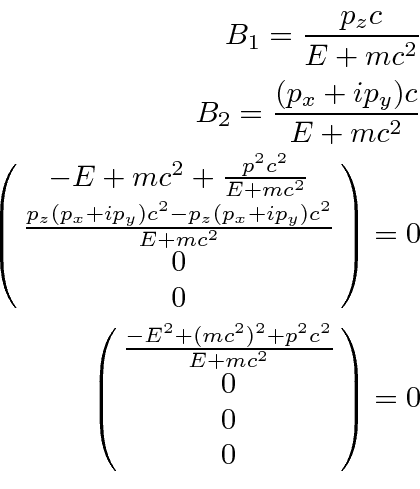This will be a solution as long as, not a surprising condition. Adding a normalization factor, the solution is.This reduces to the spin up positive energy solution for a particle at rest as the momentum goes to zero. We can therefore identify this as that same solution boosted to have momentum. The full solution is.We again see that for a non-relativistic electron, the last two components are small compared to the first. This solution is that for a positive energy electron. The fact that the last two components are non-zero does not mean it contains negative energy'' solutions.

If we make the upper two components those of a spin down electron, we get the next solution following the same procedure.This reduces to the spin down positive energy solution for a particle at rest as the momentum goes to zero. The full solution is.Now we take a look at the negative energy'' spin up solution in the same way.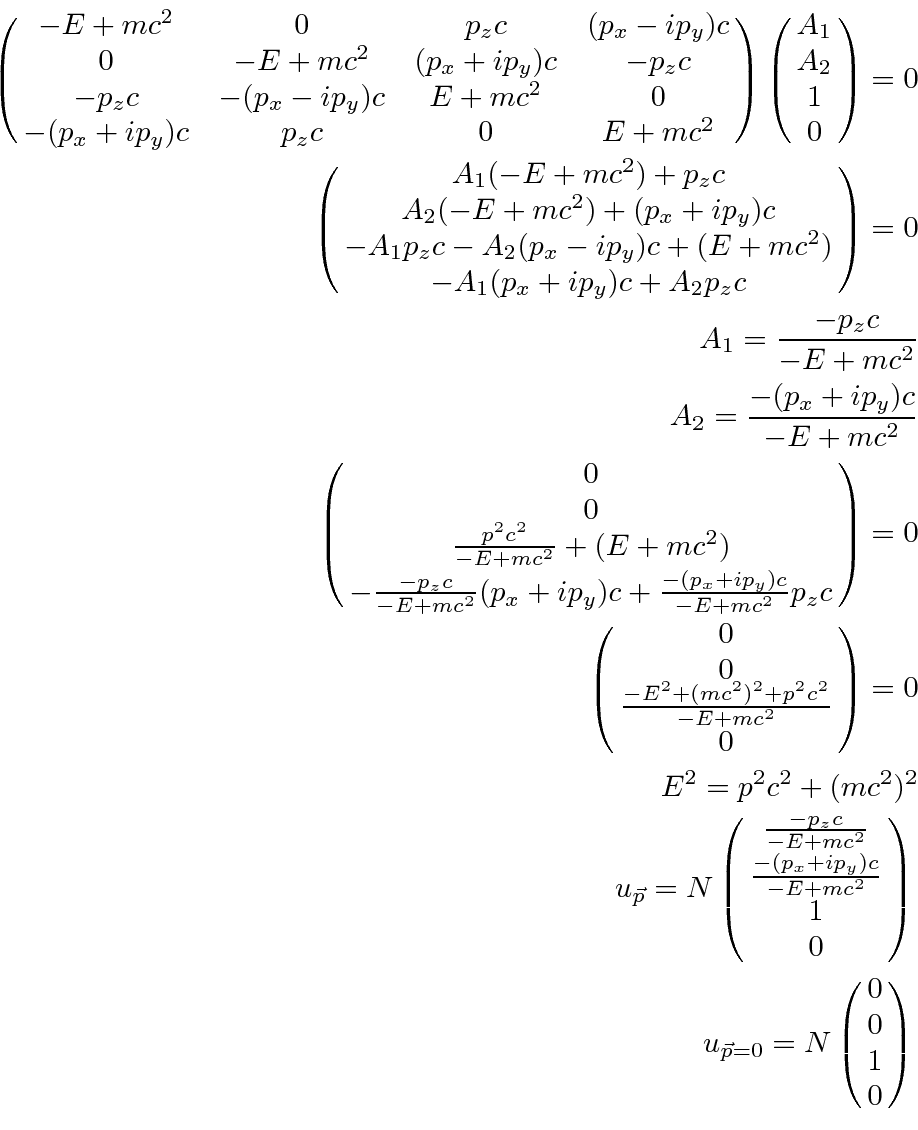This reduces to the spin up negative energy'' solution for a particle at rest as the momentum goes to zero. The full solution iswithbeing a negative number. We will eventually understand the negative energy'' solutions in terms of anti-electrons otherwise known as positrons.

Finally, the negative energy'', spin down solution follows the same pattern.withbeing a negative number.

We will normalize the states so that there is one particle per unit volume.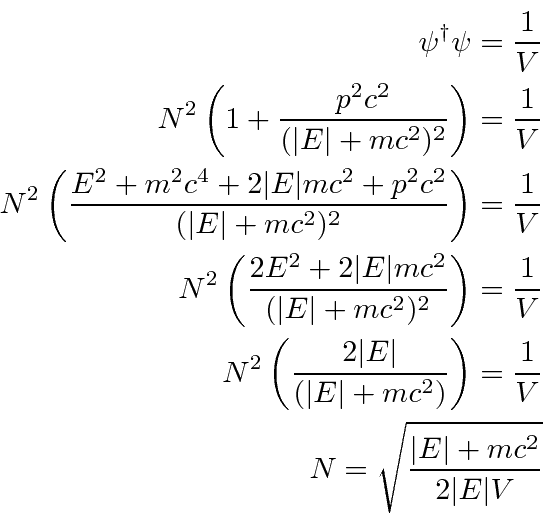We have the four solutions with for a free particle with momentum. For solutions 1 and 2,is a positive number. For solutions 3 and 4,is negative.The spinors are orthogonal for states with the same momentum and the free particle waves are orthogonal for different momenta. Note that the orthogonality condition is the same as for non-relativistic spinorsIt is useful to write the plane wave states as a spinortimes an exponential. Sakurai picks a normalization of the spinor so that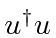transforms like the fourth component of a vector. We will follow the same convention.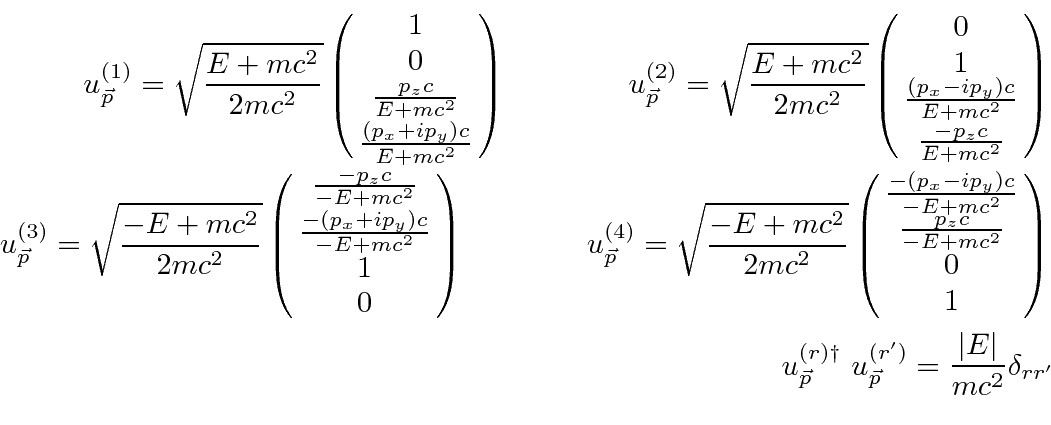Are the free particle states still eigenstates ofas were the states of a particle at rest? In general, they are not. To have an eigenvalue of +1, a spinor must have zero second and fourth components and to have an eigenvalue of -1, the first and third components must be zero. So boosting our Dirac particle to a frame in which it is moving, mixes up the spin states.

There is one case for which these are still spin eigenstates. If the particle's momentum is in the z direction, then we have just the spinors we need to be eigenstates of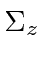. That is, if we boost along the quantization axis, the spin eigenstates are preserved. These are called helicity eigenstates. Helicity is the spin component along the direction of the particle. While it is possible to make definite momentum solutions which are eigenstates of helicity, it is not possible to make definite momentum states which are eigenstates of spin along some other direction (except in the trivial case of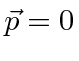as we have shown).

To further understand these solutions, we can compute the conserved probability current. First, compute it in general for a Dirac spinor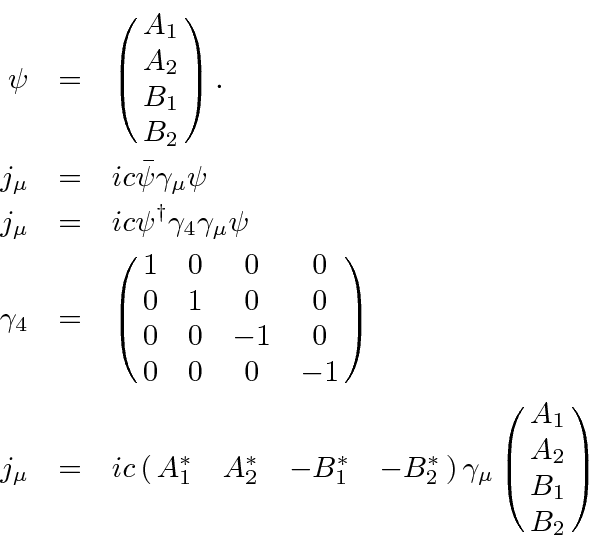Now compute it specifically for a positive energy plane wave,, and a negative energy'' plane wave,.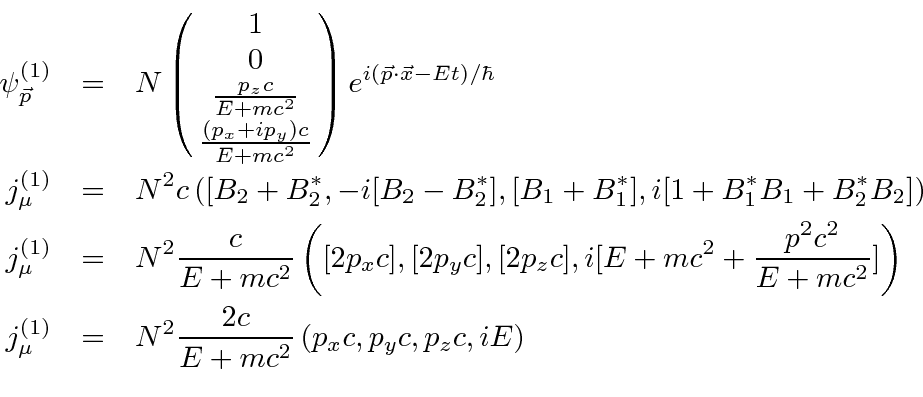Sinceis negative for the negative energy'' solution, the probability density is positive but the probability flux is in the opposite direction of the momentum.

Jim Branson 2013-04-22